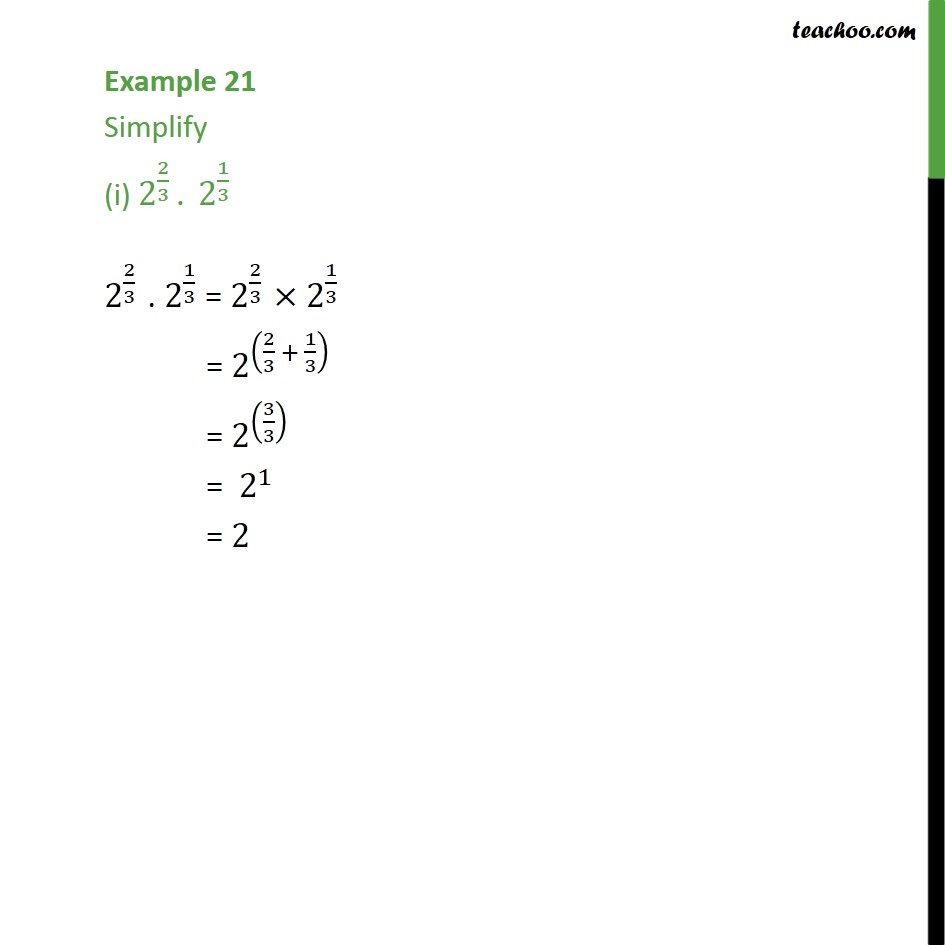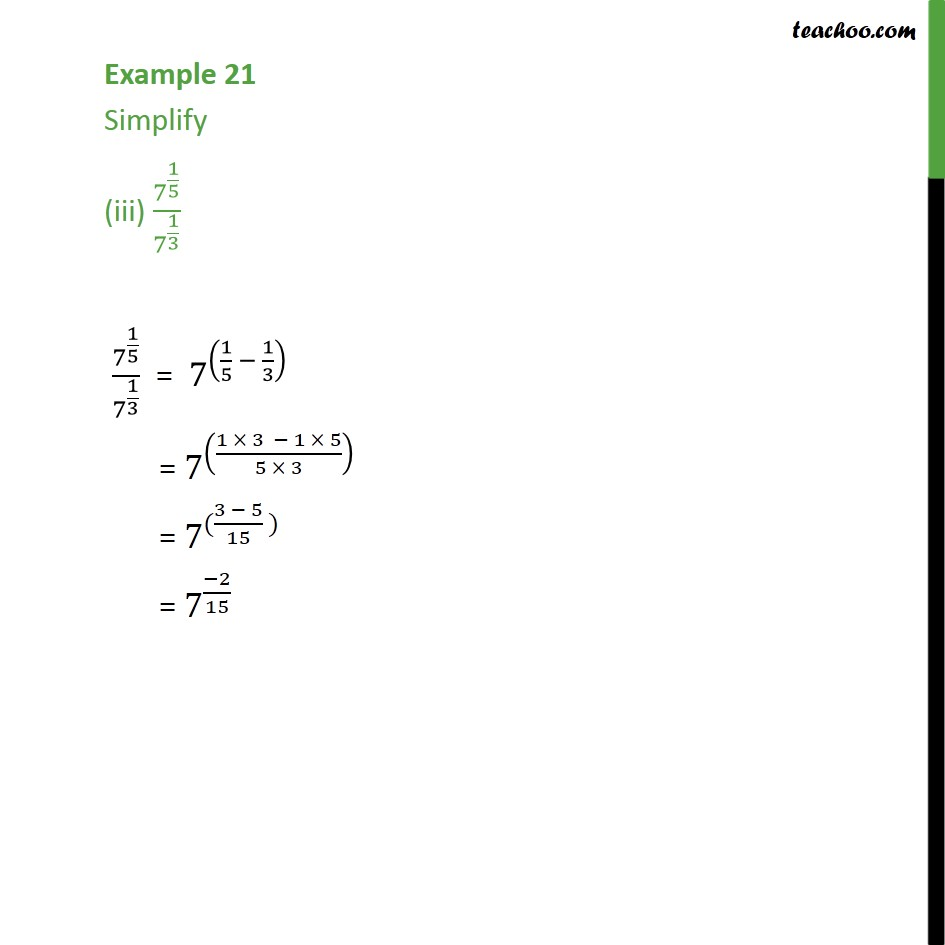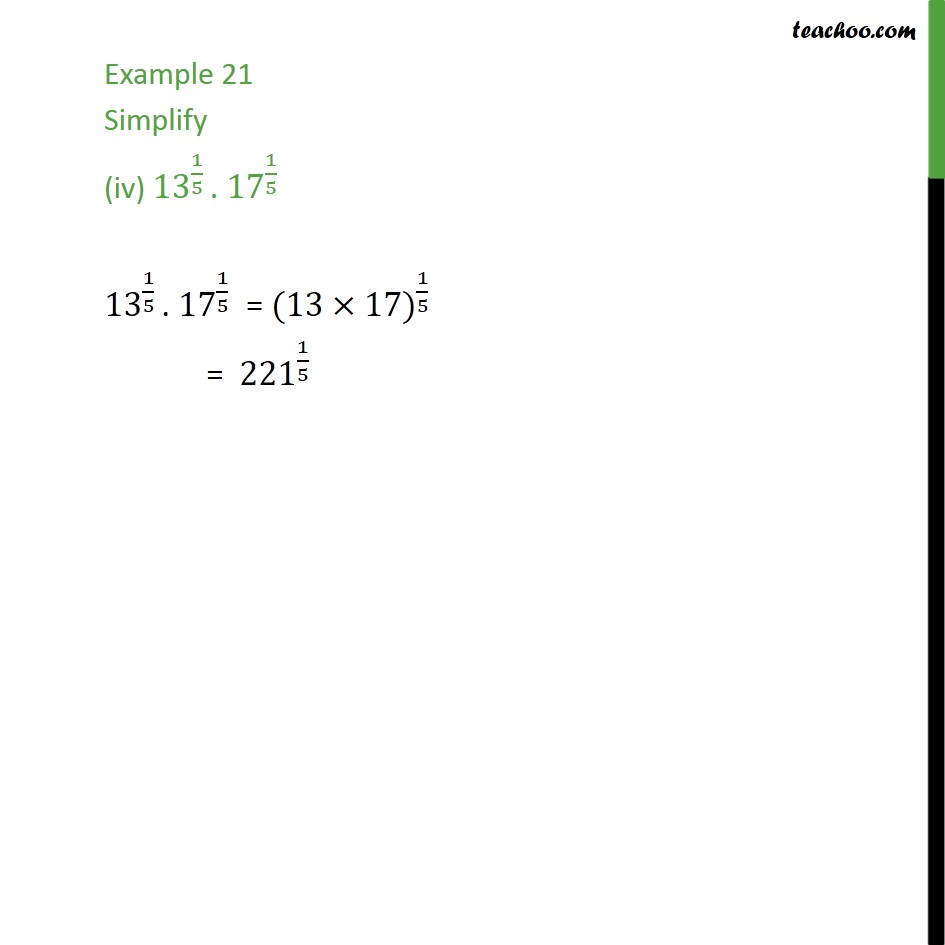Learn All Concepts of Chapter 1 Class 9 - FREE. Check - Chapter 1 Class 9 Maths1. Chapter 1 Class 9 Number Systems
2. Serial order wise
3. Examples

Transcript

Example 21 Simplify (i) 2^(2/3) . 2^(1/3) 2^(2/3) . 2^(1/3) = 2^(2/3) × 2^(1/3) = 2^((2/3 + 1/3) ) = 2^((3/3) ) = 〖 2〗^1 = 2 Example 21 Simplify (ii) (3^(1/5) )4 (3^(1/5) )^4 = 3^(1/5 × 4) = 3^(4/5) Example 21 Simplify (iii) 7^(1/5)/7^(1/3) 7^(1/5)/7^(1/3) = 7^((1/5 − 1/3) ) = 7^(((1 × 3 − 1 × 5)/(5 × 3)) ) = 7^(((3 − 5)/15 )) =〖 7〗^((−2)/15) Example 21 Simplify (iv) 〖13〗^(1/5) . 〖17〗^(1/5) 〖13〗^(1/5) . 〖17〗^(1/5) = 〖(13×17)〗^(1/5) = 〖221〗^(1/5)

Examples

About the AuthorDavneet Singh
Davneet Singh is a graduate from Indian Institute of Technology, Kanpur. He has been teaching from the past 9 years. He provides courses for Maths and Science at Teachoo.